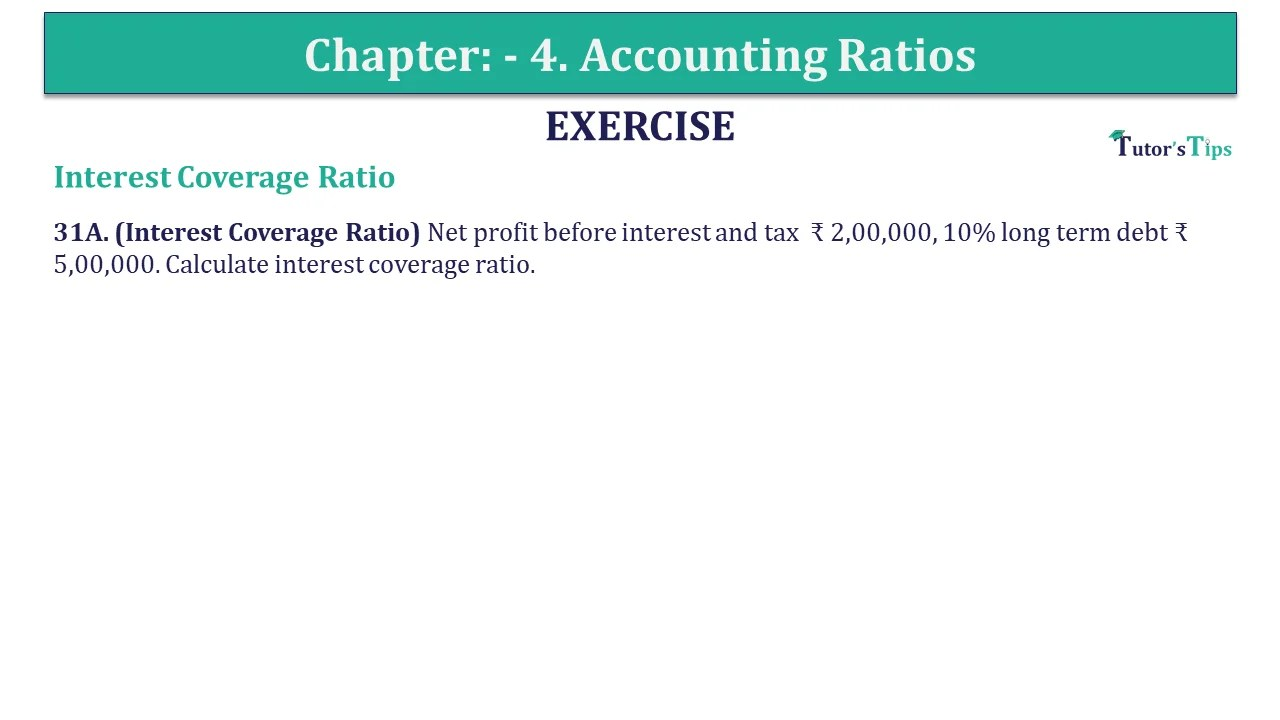# Question 31 A Chapter 4 of +2-B – USHA Publication 12 ClassQuestion 31 A Chapter 4 of +2-B

II. Solvency (Long-Term) Ratio

31A. (Interest Coverage Ratio) Net profit before interest and tax ₹ 2,00,000, 10% long term debt ₹ 5,00,000. Calculate interest coverage ratio.

## The solution of Question 31 A Chapter 4 of +2-B: –

 Interest Coverage Ratio = Net profit before interest and tax Interest
 Proprietary Ratio = ₹ 2,00,000 ₹ 50,000* = 4 times

 * Interest = 10% Interest on Long term debt = ₹ 5,00,000 X 10 100 * Interest = ₹ 50,000

What are Liquidity Ratios – Formulas and Examples

Comment if you have any question.

Also, Check out the solved question of previous Chapters: –